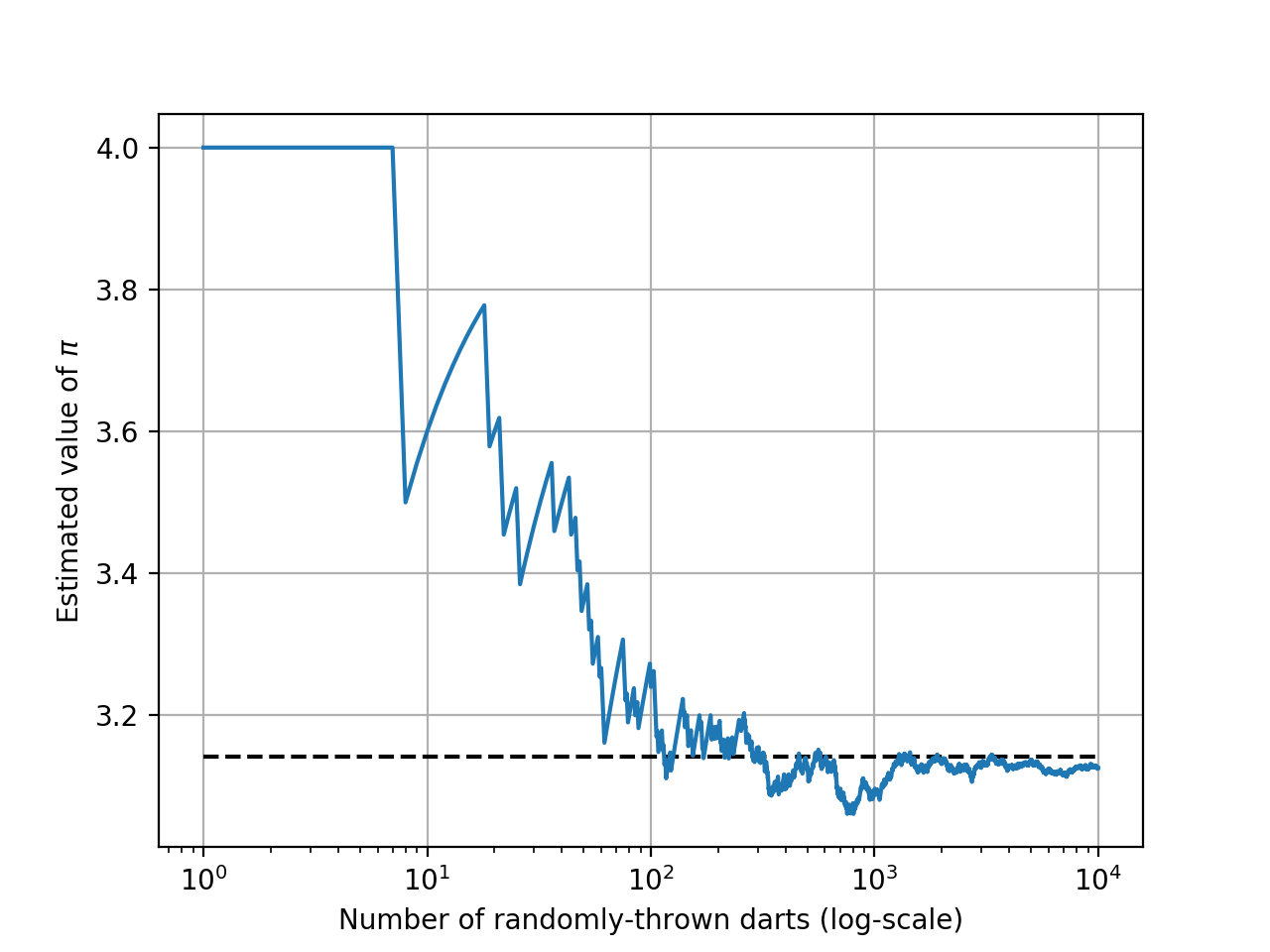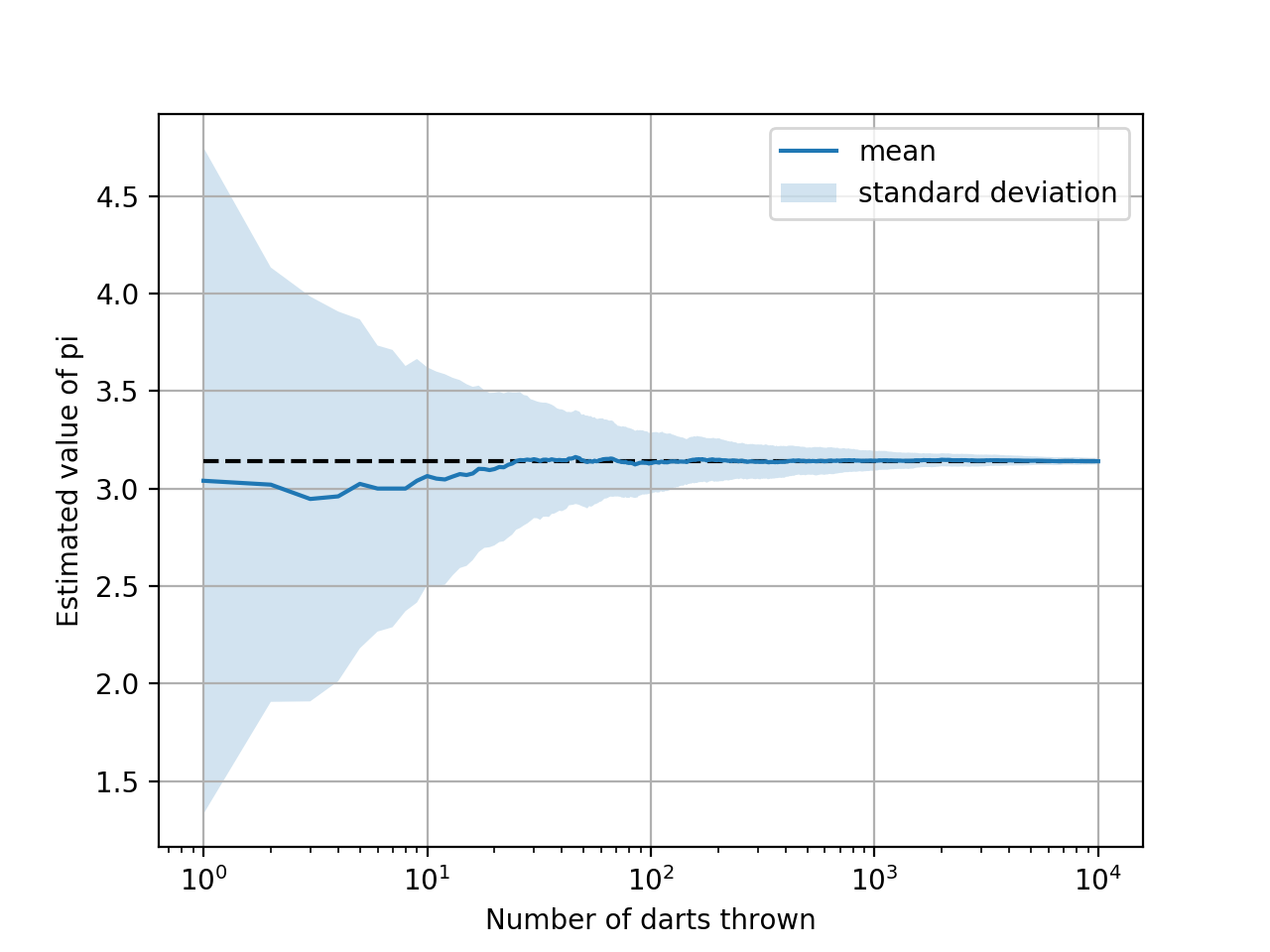# 掷镖游戏和约算Pi¶\begin{equation} P_{circle} = \frac{Area_{circle}}{Area_{square}} = \frac{\pi r^2}{(2r)^2} \end{equation}

\begin{equation} \frac{N_{circle}}{N_{total}} \approx \frac{\pi r^2}{(2r)^2} = \frac{\pi}{4} \end{equation}

## 问题1¶

\begin{equation} \sqrt{x^2 + y^2} < 1 \end{equation}

## 解（未矢量化）¶

:

import numpy as np
N = 10000
dart_positions = 2 * np.random.rand(N, 2) - 1  # 生成 [-1, 1] 中的数字


:

Ncircle =  # 将首位值设为0来简化循环中的逻辑

for x,y in dart_positions:
if np.sqrt(x**2 + y**2) < 1:
Ncircle.append(Ncircle[-1] + 1) # 镖落入了圆中
else:
Ncircle.append(Ncircle[-1])  # 镖落入了圆外——Ncircle没有变化


:

running_estimate = []

for n_total, n_circle in enumerate(Ncircle[1:]): # 跳过第一个的零值
# n_total将从0开始，所以我们需要加1
running_estimate.append(4 * n_circle / (n_total + 1))


:

running_estimate[:10]

:

[4.0, 4.0, 4.0, 4.0, 4.0, 4.0, 4.0, 4.0, 4.0, 4.0]

:

running_estimate[-10:]

:

[3.1380242217996197,
3.1381104883907125,
3.1381967377164015,
3.1378827296377825,
3.1379689844922463,
3.1380552220888354,
3.137741322396719,
3.1378275655131027,
3.137913791379138,
3.138]


## 解（矢量化）¶

:

import numpy as np
N = 10000
dart_positions = 2 * np.random.rand(N, 2) - 1  # 生成在 [-1, 1] 中的数字


:

dist_from_origin = np.sqrt((dart_positions**2).sum(axis=1))  # 形状为 (N,) 的数组


:

is_in_circle = dist_from_origin < 1  # 形状为 (N,) 的布尔数组


:

# 累计和：num_in_circle[i] = sum(is_in_circle[:i])
num_in_circle = np.cumsum(is_in_circle)

num_thrown = np.arange(1, N+1) # 1, 2, ..., N


:

running_estimate = 4 * num_in_circle / num_thrown


:

import matplotlib.pyplot as plt
%matplotlib notebook

fig, ax = plt.subplots()

ax.plot(num_thrown, running_estimate);
ax.hlines(y=np.pi, xmin=1, xmax=N+1, linestyles="--")  # 代表pi真实值的横线
ax.set_xscale("log")

# 译者注：无法翻译轴标注，因为matplotlib不支持中文字体
ax.set_ylabel(r"Estimated value of $\pi$")
ax.set_xlabel("Number of randomly-thrown darts (log-scale)")
ax.grid(True)## 解¶

:

import numpy as np

M = 100
N = 10000

dart_positions = np.random.rand(M, N, 2) * 2 - 1               # 形状为 (M, N, 2) 的位置数组
dist_from_origin = np.sqrt((dart_positions**2).sum(axis=2))    # 形状为 (M, N) 的距离数组
is_in_circle = dist_from_origin <= 1                           # 形状为 (M, N) 的布尔数组

num_thrown = np.arange(1, N+1)  # 1, 2, ..., N, 形状为 (N,)
num_in_circle = np.cumsum(is_in_circle, axis=1)  # 形状为 (M, N)

# 广播相除来产生M次测试中的pi约值
running_estimate = 4 * num_in_circle / num_thrown


:

mean_in_circled = running_estimate.mean(axis=0)  # 不同测试的平均值
std_in_circle = running_estimate.std(axis=0)     # 不同测试的标准差


:

import matplotlib.pyplot as plt
%matplotlib notebook

fig, ax = plt.subplots()

ax.plot(num_thrown, mean_in_circled, label="mean");
ax.fill_between(num_thrown, y1=mean_in_circled-std_in_circle, y2=mean_in_circled+std_in_circle,
alpha=0.2, label="standard deviation")
ax.hlines(y=np.pi, xmin=1, xmax=N+1, linestyles="--")

ax.set_xscale("log")
ax.grid(True)
ax.set_ylabel("Estimated value of pi")
ax.set_xlabel("Number of darts thrown")
ax.legend();